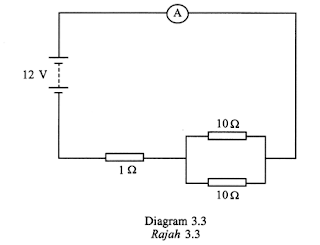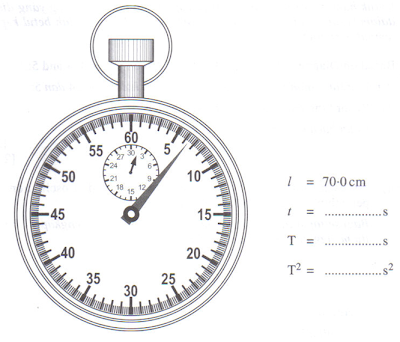## Sunday, June 7, 2009

### Paper 2 2008 No. 4

4. Diagram 4.1 shows a computer battery charger connected to a 240 Va.c. power supply. The battery charger contains box X and box Y.(a) The function of the component in box X is to lower the voltage from 240 V a.c to 20 V a.c.

(i) Name the component in box X.

……………………………………… [1 mark]

(ii) Explain why a.c and not d.c is supplied in Diagram 4.1.

……………………………………….................................................................

……………………………………….................................................................

[1 mark]

(iii) State the physics concept which explains how the component in box X works.

………………………………………. [1 mark]

(b) The efficiency of the component in box X is 80%. Calculate the input current when the output power is 65 W.
[2 marks]

(c) Box Y contains four identified components to change a.c to d.c. On Diagram 4.2, draw the electronic components in the coloured circles provided.### Paper 2 2008 No. 3

3. Diagram 3.1 and Diagram 3.2 show two photographs of electrical circuits.(a) Which diagram shows a parallel circuit?

…………………………………………… [1 mark]

(b) Draw an electrical circuit diagram for the photograph in Diagram 3.1.

[2 marks]

(c) Diagram 3.3 shows an electrical circuit. Assume that the internal resistance of the battery is negligible.(i) Calculate the effective resistance of the circuit.

[2 marks]

(ii) What is the reading of the ammeter?

[1 mark]

(iii) One of the 10 Ω resistors is removed from the circuit. What happens to the ammeter reading?

………………………………………. [1 mark]

### Paper 2 2008 No. 2

2. A pencil is placed in front of a concave mirror. Diagram 2.1 shows the image of the pencil in the mirror.(a) Name the phenomenon involved in the formation of the image.

……………………………………………
[1 mark]

(b) The image is upright and magnified. State one other characteristic of the image.

……………………………………………
[1 mark]

(c) Diagram 2.2 shows an incomplete ray diagram. C is the centre of curvature and F is the principle focus. Complete the ray diagram to show how the image is formed.### Paper 2 2008 No. 1

1. Diagram 1 shows a slinky spring being moved left and right continuously.(a) Complete the sentence below by ticking (x) the correct box.
The wave produced by the slinky is a
Transverse wave -------
Longitudinal wave -------
[1 mark]

(b) On Diagram 1, mark ‘X’ on any one of the crests of the wave.
[1 mark]

(c) Complete the following sentence by underlining the correct phrase in the bracket.

The direction of the hand movement is (the same as / opposite to / perpendicular to ) the direction of the wave movement.
[1 mark]

(d) What is transferred by the wave?

……………………………………………
[1 mark]

## Saturday, June 6, 2009

### SPM 2008 Paper 3 No. 1

A students carries out an experiment to investigate the relationship between the length, l , of a simple pendulum and the period of oscillation, T. The apparatus set-up for this experiment is shown in Diagram 1.The student starts the experiment with a pendulum length of 30.0 cm. The pendulum bob is displaced and then released so that it oscillates. The time taken, t, for 10 complete oscillations is recorded. The experiment is repeated with pendulum lengths of 40.0 cm, 50.0 cm, 60.0 cm and 70.0 cm.
The corresponding stopwatch readings for 10 complete oscillations are shown in Diagram 5.2, 5.3, 5.4 , 5.5 and 5.6.(a) For the experiment described on pages6, 7and 8, identify:

(i) The manipulated variable

………………………………………………………………………………………..[1 mark]

(ii) The responding variable

……………………………………………………………………………………...[1 mark]

(iii) The constant variable

……………………………………………………………………………………...[1 mark]

(b) For this part of question, write your answers in the spaces provided in the corresponding Diagrams. Your answers must be correct to two decimal places.
Based on Diagrams 5.2, 5.3, 5.4, 5.5 and 5.6:
(i) Record the readings, t, of the stopwatch. [2 marks]

(ii) For each value of t in 5(b)(i), calculate the period of oscillation, T, of the pendulum using the following equation:
T = t/10
Record the value of T. [1 mark]

(iii) Calculate T2 for each value of T in 5(b)(ii). Record the value of T2. [2 marks]

(c) Tabulate your results for all values of l, T and T2 in the space below.

[2 marks]

(d) On the graph paper on page10, plot a graph of T2 against l. [5 marks]

(e) Based on your graph in 5(d), state the relationship between T2 and l.

……………………………………………………………………………………….[1 mark]

Responding variable: Time for 10 complete oscillation/ t / Period / T
Constant variable : mass of bob // gravitational acceleration1. Show T2 on the vertical –axis (y-axis) and l on the horizontal – axis (x-axis)
2. State the units of the variables correctly
3. Both axes are marked with uniform scale
4. All five points are plotted correctly
5. Best fit line is drawn
6. Show the minimum size of graph at least 5 x 4 (2 cm x 2 cm) squares

T2 is directly proportional to l

## Monday, December 1, 2008

### Spm 2005: Paper 2, Question No. 2:

Two similar loudspeaker are placed 0.8 m apart. They emit a coherent sound wave. A girl walks slowly parallel to the two loudspeakers at a distance 3.2 m in front of the loudspeakers. She hears a loud sound and weak sound alternately. Figure 2.1 shows the positions of the loud and weak sounds.(a) What is meant by a coherent wave?



(b) The distance between three positions of the loud sound is 4.5 m, as shown in Figure 2.1.

Calculate the wavelength of the sound wave.



(c) The loud sound is heard due to the constructive interference of the sound waves. State how the constructive interference occurs.